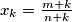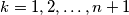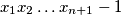### IMO Shortlist 2015 problem N3

Kvaliteta:
Avg: 0,0
Težina:
Avg: 7,0

Let$m$ and$n$ be positive integers such that$m>n$. Define$x_k=\frac{m+k}{n+k}$ for$k=1,2,\ldots,n+1$. Prove that if all the numbers$x_1,x_2,\ldots,x_{n+1}$ are integers, then$x_1x_2\ldots x_{n+1}-1$ is divisible by an odd prime.

(Austria)

Izvor: https://www.imo-official.org/problems/IMO2015SL.pdf